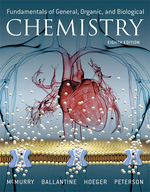×
Get Full Access to Fundamentals Of General, Organic, And Biological Chemistry (Mastering Chemistry) - 8 Edition - Chapter 1 - Problem 1.85
Get Full Access to Fundamentals Of General, Organic, And Biological Chemistry (Mastering Chemistry) - 8 Edition - Chapter 1 - Problem 1.85

×ISBN: 9780134015187 2044

## Solution for problem 1.85 Chapter 1

Fundamentals of General, Organic, and Biological Chemistry (Mastering Chemistry) | 8th Edition

• Textbook Solutions
• 2901 Step-by-step solutions solved by professors and subject experts
• Get 24/7 help from StudySoup virtual teaching assistantsFundamentals of General, Organic, and Biological Chemistry (Mastering Chemistry) | 8th Edition

4 5 1 319 Reviews
22
3
Problem 1.85

When 418 J of heat is applied to a 125 g sample, the temperature increases by 28$${ }^{\circ} \mathrm{C}$$. Calculate the specific heat of the sample and compare your answer to the values in Table 1.10. What is the identity of the sample?

Text Transcription:

^circ C

Step-by-Step Solution:
Step 1 of 3

September 29,2017 – Lecture 11  Metals – lose electrons from their valence shells. o Forms positive ions, cations o Lustrous (shiny), malleable, ductile, and are good conductors of heat and electricity o They are mostly solids at room temperature (except Mercury – liquid at room temperature)  Nonmetals – add electrons to their valence shells. o Form negative ions, anions o Share electrons with metalloids or other nonmetals o Very electronegative o Dull, brittle, nonconductors (insulators) o Some are solid, but many are gases, and Bromine is a liquid  Metalloids – metalloids, aka semi-metals o They have characteristics of both metals and nonmetals o They are shiny but brittle o They are semiconductors  Metallic behavior in group 5A(15) and Period 3: o Metallic behavior decreases across the period o Metallic behavior increases down the group Question #1: Which pair of elements has the less metallic element listed first a) As, Sb b) Si, P c) Na, Be d) Cs, Rn e) Sn, Te Question #2: Which of the following elements would you expect to be the most metallic a) In b) Si c) Ca d) Sr e) Al  Oxides: molecules with O 2- o Metallic elements have basic oxides o Nonmetallic elements have acidic oxides  Metal halides are ionic, nonmetallic, halides are covalent  More metallic elements react with weaker acids (H O)

Step 2 of 3

Step 3 of 3

## Discover and learn what students are asking

Calculus: Early Transcendental Functions : Iterated Integrals and Area in the Plane
?In Exercises 1 - 10, evaluate the integral. $$\int_{0}^{\sqrt{4-x^{2}}} x^{2} y d y$$

Chemistry: The Central Science : Liquids and Intermolecular Forces
?The phase diagram for neon is Use the phase diagram to answer the following

Statistics: Informed Decisions Using Data : Inference about the Difference between Two Medians: Dependent Samples
?In Problems 3–10, use the Wilcoxon matched-pairs signedranks test to test the given hypotheses at the a = 0.05 level of significance. The dependent sa

Statistics: Informed Decisions Using Data : Inference about the Difference between Two Medians: Dependent Samples
?Does Octane Affect Miles per Gallon? A researcher wants to know if the octane level of gasoline affects the gas mileage of a car. She randomly selects

Unlock Textbook Solution